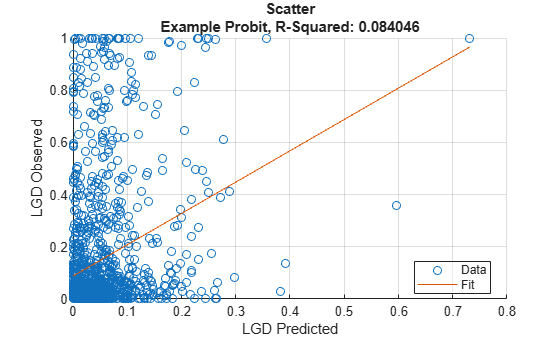# Regression

Create `Regression` model object for loss given default

Since R2021a

## Description

Create and analyze a `Regression` model object to calculate the loss given default (LGD) using this workflow:

1. Use `fitLGDModel` to create a `Regression` model object.

2. Use `predict` to predict the LGD.

3. Use `modelDiscrimination` to return AUROC and ROC data. You can plot the results using `modelDiscriminationPlot`.

4. Use `modelCalibration` to return the R-square, RMSE, correlation, and sample mean error of the predicted and observed LGD data. You can plot the results using `modelCalibrationPlot`.

## Creation

### Syntax

``RegressionLGDModel = fitLGDModel(data,ModelType)``
``RegressionLGDModel = fitLGDModel(___,Name,Value)``

### Description

example

````RegressionLGDModel = fitLGDModel(data,ModelType)` creates a `Regression` LGD model object.```

example

````RegressionLGDModel = fitLGDModel(___,Name,Value)` specifies options using one or more name-value pair arguments in addition to the input arguments in the previous syntax. The optional name-value pair arguments set model object properties. For example, ```lgdModel = fitLGDModel(data,'regression','PredictorVars',{'LTV' 'Age' 'Type'},'ResponseVar','LGD','ResponseTransform','probit','BoundaryTolerance',1e-6)``` creates a `lgdModel` object using a `Regression` model type. ```

### Input Arguments

expand all

Data for loss given default, specified as a table where the first column and all other columns except the last column are `PredictorVars`, the last column is `ResponseVar`.

Data Types: `table`

Model type, specified as a string with the value of `"Regression"` or a character vector with the value of `'Regression'`.

Data Types: `char` | `string`

Name-Value Arguments

Specify optional pairs of arguments as `Name1=Value1,...,NameN=ValueN`, where `Name` is the argument name and `Value` is the corresponding value. Name-value arguments must appear after other arguments, but the order of the pairs does not matter.

Before R2021a, use commas to separate each name and value, and enclose `Name` in quotes.

Example: ```lgdModel = fitLGDModel(data,'regression','PredictorVars',{'LTV' 'Age' 'Type'},'ResponseVar','LGD','ResponseTransform','probit','BoundaryTolerance',1e-6)```

User-defined model ID, specified as the comma-separated pair consisting of `'ModelID'` and a string or character vector. The software uses the `ModelID` text to format outputs and is expected to be short.

Data Types: `string` | `char`

User-defined description for model, specified as the comma-separated pair consisting of `'Description'` and a string or character vector.

Data Types: `string` | `char`

Predictor variables, specified as the comma-separated pair consisting of `'PredictorVars'` and a string array or cell array of character vectors. `PredictorVars` indicates which columns in the `data` input contain the predictor information. By default, `PredictorVars` is set to all the columns in the `data` input except for the `ResponseVar`.

Data Types: `string` | `cell`

Response variable, specified as the comma-separated pair consisting of `'ResponseVar'` and a string or character vector. The response variable contains the LGD data and must be a numeric variable. An LGD value of `0` indicates no loss (full recovery), `1` indicates total loss (no recovery), and values between `0` and `1` indicate a partial loss. By default, the `ResponseVar` is set to the last column of `data`.

Data Types: `string` | `char`

Boundary tolerance, specified as the comma-separated pair consisting of `'BoundaryTolerance'` and a positive scalar numeric. The `BoundaryTolerance` value perturbs the LGD response values away from 0 and 1, before applying a `ResponseTransform`.

Data Types: `double`

Response transform, specified as the comma-separated pair consisting of `'ResponseTransform'` and a character vector or string.

Data Types: `string` | `char`

## Properties

expand all

User-defined model ID, returned as a string.

Data Types: `string`

User-defined description, returned as a string.

Data Types: `string`

Underlying statistical model, returned as a compact linear model object. The compact version of the underlying regression model is an instance of the `classreg.regr.CompactLinearModel` class. For more information, see `fitlm` and `CompactLinearModel`.

Data Types: `CompactLinearModel`

Predictor variables, returned as a string array.

Data Types: `string`

Response variable, returned as a scalar string.

Data Types: `string`

Boundary tolerance, returned as a scalar numeric.

Data Types: `double`

Response transform, returned as a string.

Data Types: `string`

## Object Functions

 `predict` Predict loss given default `modelDiscrimination` Compute AUROC and ROC data `modelDiscriminationPlot` Plot ROC curve `modelCalibration` Compute R-square, RMSE, correlation, and sample mean error of predicted and observed LGDs `modelCalibrationPlot` Scatter plot of predicted and observed LGDs

## Examples

collapse all

This example shows how to use `fitLGDModel` to create a `Regression` model for loss given default (LGD).

```load LGDData.mat head(data)```
``` LTV Age Type LGD _______ _______ ___________ _________ 0.89101 0.39716 residential 0.032659 0.70176 2.0939 residential 0.43564 0.72078 2.7948 residential 0.0064766 0.37013 1.237 residential 0.007947 0.36492 2.5818 residential 0 0.796 1.5957 residential 0.14572 0.60203 1.1599 residential 0.025688 0.92005 0.50253 investment 0.063182 ```
```rng('default'); NumObs = height(data); c = cvpartition(NumObs,'HoldOut',0.4); TrainingInd = training(c); TestInd = test(c);```

Create `Regression` LGD Model

Use `fitLGDModel` to create a `Regression` model using the `TrainingInd` data.

```lgdModel = fitLGDModel(data(TrainingInd,:),'regression',... 'ModelID','Example Probit',... 'Description','Example LGD probit regression model.',... 'PredictorVars',{'LTV' 'Age' 'Type'},... 'ResponseVar','LGD','ResponseTransform','probit','BoundaryTolerance',1e-6); disp(lgdModel)```
``` Regression with properties: ResponseTransform: "probit" BoundaryTolerance: 1.0000e-06 ModelID: "Example Probit" Description: "Example LGD probit regression model." UnderlyingModel: [1x1 classreg.regr.CompactLinearModel] PredictorVars: ["LTV" "Age" "Type"] ResponseVar: "LGD" ```

Display the underlying model. The underlying model's response variable is the probit transformation of the LGD response data. Use the `'ResponseTransform'` and `'BoundaryTolerance'` arguments to modify the transformation.

`lgdModel.UnderlyingModel`
```ans = Compact linear regression model: LGD_probit ~ 1 + LTV + Age + Type Estimated Coefficients: Estimate SE tStat pValue ________ ________ _______ __________ (Intercept) -2.2212 0.14824 -14.984 2.7409e-48 LTV 1.192 0.17183 6.9372 5.3152e-12 Age -0.60356 0.035256 -17.119 1.3828e-61 Type_investment 0.61814 0.1018 6.0723 1.4933e-09 Number of observations: 2093, Error degrees of freedom: 2089 Root Mean Squared Error: 1.74 R-squared: 0.197, Adjusted R-Squared: 0.196 F-statistic vs. constant model: 171, p-value = 4.39e-99 ```

Predict LGD

For LGD prediction, use `predict`. The LGD model applies the inverse transformation so the predictions are in the LGD scale, not in the transformed scale used to fit the underlying model.

`predictedLGD = predict(lgdModel,data(TestInd,:))`
```predictedLGD = 1394×1 0.0011 0.0058 0.2091 0.0015 0.0192 0.0620 0.0757 0.0000 0.0156 0.0388 ⋮ ```

Validate LGD Model

Use `modelDiscriminationPlot` to plot the ROC curve.

`modelDiscriminationPlot(lgdModel,data(TestInd,:))`Use `modelCalibrationPlot` to show a scatter plot of the predictions.

`modelCalibrationPlot(lgdModel,data(TestInd,:))`